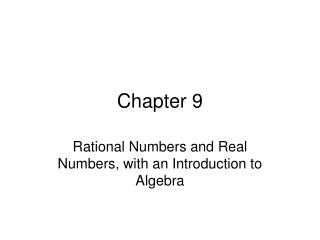DownloadDownload PresentationChapter 9

# Chapter 9

Download Presentation## Chapter 9

- - - - - - - - - - - - - - - - - - - - - - - - - - - E N D - - - - - - - - - - - - - - - - - - - - - - - - - - -
##### Presentation Transcript

1. Chapter 9 Rational Numbers and Real Numbers, with an Introduction to Algebra

2. 9.1 The Rational Numbers The setof rational numbers is the set

3. Definition: Equality or Rational Numbers if and only if Theorem: Let be any rational number and n any nonzero integer. Then

4. Simplest Form A rational number is said to be in simplest form (lowest terms) when its numerator and denominator have no common prime factors, and its denominator is positive.

5. Addition of Rational Numbers Definition: Let and be any rational numbers. Then Theorem: Let be any rational number. Then

6. Properties of Rational Numbers • Closure Property of Addition. • Commutative Property of Addition • Associative Property of Addition • Additive Identity Property • Additive Inverse Property

7. Theorem: Let be any rational numbers. If then Theorem: Let be any rational number. Then

8. Subtraction Definition: Let and be any rational numbers. Then

9. Multiplication Definition:Let and be any rational numbers. Then

10. Properties of Rational Number Multiplication • Closure Property of Multiplication. • Commutative Property of Multiplication. • Associative Property of Multiplication • Multiplicative Identity Property • Multiplicative Inverse Property

11. Division Definition: Let and be any rational numbers where Then

12. Three Methods of Rational-Number Division Let and be any rational numbers where Then the following are equivalent.

13. Cross Multiplication of Rational Number Inequality Let and be any rational numbers, where and Then

14. 9.2 The Real Numbers Theorem:There is no rational number whose square is 2.

15. Definition: The set of real numbers, R, is the set of all numbers that have an infinite decimal representation. Real Numbers (Decimals) Irrational Numbers (nonterminating, nonrepeating decimals) Rational Numbers Terminating Decimals Nonterminating Decimals

16. Definition: Let a be a nonnegative real number. Then the principal square root of a is defined as Definition: Let a be a real number and n a positive integer. • If then if and only if • If and n is odd, then if and only if where

17. Rational Exponents Let a be any real number and n any positive integer. Then Let a be a nonnegative number and be a rational number in simplest form. Then

18. Exponent Rules

19. Introduction to Algebra • An equation is a mathematical statement. • A solution is a number that makes the mathematical statement true. • The set of all solutions is called a solution set. • An inequality is a mathematical statement using the phrase “less than” or “greater than” or “less than or equal to”, etc.